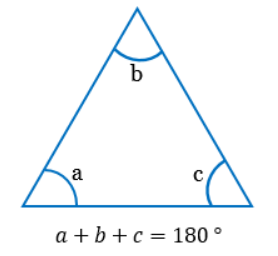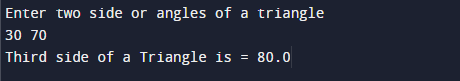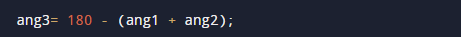C program to enter two angles of a triangle and find the third angle.

In this tutorial you will learn about the C program to enter two angles of a triangle and find the third angle. and its application with practical example.

In this tutorial, we will learn to create a C program that will find side of a Triangle.If two side of a Triangle are given   using C programming.

Prerequisites

Before starting with this tutorial we assume that you are best aware of the following C programming topics:

• Arithmetic operators
• Data types
• Basic input/output.

Basic Properties of triangle

The sum of the interior angle on the same vertex is 180°C Program to enter two angles of a triangle and find the third angle.

Here we take two side of a triangle and with the help of angle property we will find the required third side of a triangle ,Let’s take a look to program.

outputthe above program, we have first declared and initialized a set variables required in the program.

• ang1 =it is for holding the angle value 1.
• ang2 =it is for holding the angle value 2.
• ang3 =it is for holding the angle value 3.

One of the common property of a triangles is that all three(3) interior angles (∠a + ∠b +∠c) of a triangle on adding will give to 180 degrees. This is an important theorem of geometry also known as Triangle Angle Sum Theorem(∠a + ∠b +∠c).According to this Theorem, the sum of the three interior angles in a triangle is always 180°.

So using this triangle  angle sum theorem we create a program in which we get two angle from user and by adding them subtract from 180 -(ang1+ang2) we will get our required third angle .as we can see here by adding two given angle and subtract them from 180 we get the third required angle ..

In this tutorial we have learn about the C program to enter two angles of a triangle and find the third angle. and its application with practical example. I hope you will like this tutorial.